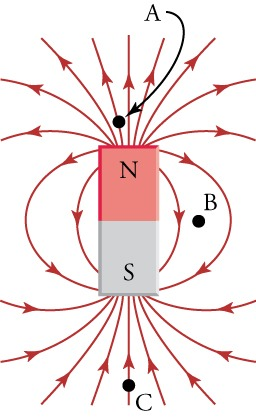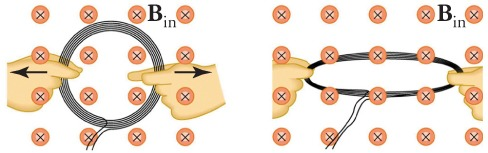Sections
Chapter Review

# Chapter Review

### Concept Items

#### 20.1Magnetic Fields, Field Lines, and Force

1.
If you place a small needle between the north poles of two bar magnets, will the needle become magnetized?
1. Yes, the magnetic fields from the two north poles will point in the same directions.
2. Yes, the magnetic fields from the two north poles will point in opposite directions.
3. No, the magnetic fields from the two north poles will point in opposite directions.
4. No, the magnetic fields from the two north poles will point in the same directions.
2. If you place a compass at the three points in the figure, at which point will the needle experience the greatest torque? Why?1. The density of the magnetic field is minimized at B, so the magnetic compass needle will experience the greatest torque at B.
2. The density of the magnetic field is minimized at C, so the magnetic compass needle will experience the greatest torque at C.
3. The density of the magnetic field is maximized at B, so the magnetic compass needle will experience the greatest torque at B.
4. The density of the magnetic field is maximized at A, so the magnetic compass needle will experience the greatest torque at A.
3.
In which direction do the magnetic field lines point near the south pole of a magnet?
1. Outside the magnet the direction of magnetic field lines is towards the south pole of the magnet.
2. Outside the magnet the direction of magnetic field lines is away from the south pole of the magnet.

#### 20.2Electromagnetic Induction

4. Yes or no—Is an emf induced in the coil shown when it is stretched? If so, state why and give the direction of the induced current.1. No, because induced current does not depend upon the area of the coil.
2. Yes, because area of the coil increases; the direction of the induced current is counterclockwise.
3. Yes, because area of the coil increases; the direction of the induced current is clockwise.
4. Yes, because the area of the coil does not change; the direction of the induced current is clockwise.
5.
What is Lenz’s law?
1. If induced current flows, its direction is such that it adds to the changes which induced it.
2. If induced current flows, its direction is such that it opposes the changes which induced it.
3. If induced current flows, its direction is always clockwise to the changes which induced it.
4. If induced current flows, its direction is always counterclockwise to the changes which induced it.
6.

Explain how magnetic flux can be zero when the magnetic field is not zero.

1. If angle between magnetic field and area vector is 0°, then its sine is also zero, which means that there is zero flux.
2. If angle between magnetic field and area vector is 45°, then its sine is also zero, which means that there is zero flux.
3. If angle between magnetic field and area vector is 60°, then its cosine is also zero, which means that there is zero flux.
4. If the angle between magnetic field and area vector is 90°, then its cosine is also zero, which means that there is zero flux.

#### 20.3Motors, Generators, and Transformers

7.
Consider the angle between the area vector and the magnetic field in an electric motor. At what angles is the torque on the wire loop the greatest?
1. $0∘$and $180∘$
2. $45∘$ and $135∘$
3. $90∘$ and $270∘$
4. $225∘$ and $315∘$
8.
What is a voltage transformer?
1. A transformer is a device that transforms current to voltage.
2. A transformer is a device that transforms voltages from one value to another.
3. A transformer is a device that transforms resistance of wire to voltage..
9.

Why is electric power transmitted at high voltage?

1. To increase the current for the transmission
2. To reduce energy loss during transmission
3. To increase resistance during transmission
4. To reduce resistance during transmission

### Critical Thinking Items

#### 20.1Magnetic Fields, Field Lines, and Force

10.

True or false—It is not recommended to place credit cards with magnetic strips near permanent magnets.

1. false
2. true
11.

True or false—A square magnet can have sides that alternate between north and south poles.

1. false
2. true
12.

You move a compass in a circular plane around a planar magnet. The compass makes four complete revolutions. How many poles does the magnet have?

1. two poles
2. four poles
3. eight poles
4. 12 poles

#### 20.2Electromagnetic Induction

13.
To obtain power from the current in the wire of your vacuum cleaner, you place a loop of wire near it to obtain an induced emf. How do you place and orient the loop?
1. A loop of wire should be placed nearest to the vacuum cleaner wire to maximize the magnetic flux through the loop.
2. A loop of wire should be placed farthest to the vacuum cleaner wire to maximize the magnetic flux through the loop.
3. A loop of wire should be placed perpendicular to the vacuum cleaner wire to maximize the magnetic flux through the loop.
4. A loop of wire should be placed at an angle greater than 90° to the vacuum cleaner wire to maximize the magnetic flux through the loop.
14.
A magneto is a device that creates a spark across a gap by creating a large voltage across the gap. To do this, the device spins a magnet very quickly in front of a wire coil, with the ends of the wires forming the gap. Explain how this creates a sufficiently large voltage to produce a spark.
1. The electric field in the coil increases rapidly due to spinning of magnet which creates an emf in the coil that is proportional to the rate of change of the magnetic flux.
2. The magnetic field in the coil changes rapidly due to spinning of magnet which creates an emf in the coil that is proportional to the rate of change of the magnetic flux.
15.
If you drop a copper tube over a bar magnet with its north pole up, is a current induced in the copper tube? If so, in what direction? Consider when the copper tube is approaching the bar magnet.
1. Yes, the induced current will be produced in the clockwise direction when viewed from above.
2. No, the induced current will not be produced.

#### 20.3Motors, Generators, and Transformers

16.
How can you maximize the peak emf from a generator?
1. The peak emf from a generator can be maximized only by maximizing number of turns.
2. The peak emf from a generator can be maximized only by maximizing area of the wired loop.
3. The peak emf from a generator can be maximized only by maximizing frequency.
4. The peak emf from a generator can be maximized by maximizing number of turns, maximizing area of the wired loop or maximizing frequency.
17.

Explain why power is transmitted over long distances at high voltages.

1. Plost = ItransmittedVtransmitted, so to maximize current, the voltage must be maximized.
2. Ptransmitted = ItransmittedVtransmitted, so to maximize current, the voltage must be maximized.
3. Plost = ItransmittedVtransmitted, so to minimize current, the voltage must be maximized.
4. Ptransmitted = ItransmittedVtransmitted, so to minimize current, the voltage must be maximized.

### Problems

#### 20.1Magnetic Fields, Field Lines, and Force

18.

A straight wire segment carries 0.25 A. What length would it need to be to exert a 4.0-mN force on a magnet that produces a uniform magnetic field of 0.015 T that is perpendicular to the wire?

1. 0.55 m
2. 1.10 m
3. 2.20 m
4. 4.40 m

#### 20.2Electromagnetic Induction

19.

What is the current in a wire loop of resistance 10 Ω through which the magnetic flux changes from zero to 10 Wb in 1.0 s?

1. –100 A
2. –2.0 A
3. –1.0 A
4. +1.0 A
20.

An emf is induced by rotating a 1,000 turn, 20.0 cm diameter coil in Earth’s 5.00 × 10–5 T magnetic field. What average emf is induced, given the plane of the coil is originally perpendicular to Earth’s field and is rotated to be parallel to the field in 10.0 ms?

1. –1.6 × 10-4 V
2. +1.6 × 10-4 V
3. +1.6 × 10-1 V
4. –1.6 × 10-1 V